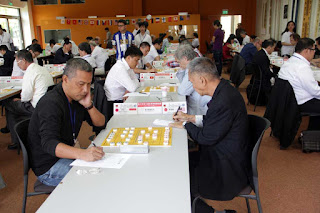## 2015 14th World Xiangqi Championship. Round 1: Lay Kam Hock (West Malaysia) wins Iwan Setiawan (Indonesia)Both players on actionLay Kim Hock (West Malaysia)Iwan Setiawan (Indonesia)

Lay Kam Hock (West Malaysia) wins Iwan Setiawan (Indonesia)

Round 1
2015 14th World Xiangqi Championship
Date: 22nd August 2015
Venue: University of Applied Languages (SDI), Munich, Germany

1. C2=5  …………

Central cannon opening.

1. …………  H8+7    2. H2+3  R9=8
3. P7+1  H2+1

In this game, Black uses Single Horse Defense against Red central cannon opening.
Normally in this situation, Black will play P7+1. This is because Single Horse defense is not a idea opening to against central cannon.

4. H8+7  C8=9    5. P3+1  R1+1
6. H7+6  …………

Good move. As seen, Black's central line is the weakness. Red H7+6 is a good move to attack Black's central pawn.

6. …………  E7+5

Black forces to consolidate his central line first.

7. C8=6  …………

Good move, prevent Black's chariot R1=4 to capture horse.

7. …………  C2+3

Black cannot allow Red's horse stays on river bank, C2+3 is to force Red to moves the horse.

8. P7+1  …………

? Red can straight away H6+5 to attack on center line.

8. …………  P3+1    9. H6+5  …………

H6+4 better.

9. …………  H7+5   10. C5+4  A4+5
11. R9=8  P3+1   12. E7+9  R1=4
13. A6+5  R4+2   14. C6=5  R4+2
15. C5-2  R4+1

Black's chariot moves too frequent. P1+1 would be better.

16. +C=8  P3=2   17. R8+4  R4=1
18. R8+2  R1+1   19. R8=3  R8+6
20. R1+2  R8=7   21. P5+1  P1+1
22. R3=6  …………

? Red gives up his pawn so easily.

22. …………  H1+2

Black should R7-1.

23. R6+2  E5-7   24. R6=7  E3+5
25. H3-1  …………

Red setting up a small trap, prepare for C5+5.

25. …………  R1+2   26. A5-6  H2-4
27. R7-2  H4+5   28. A4+5  C9=7
29. R1=2  R7+2

Good move.

30. R7=8  R7=9   31. R2+1  C7+7
32. R2=6  A5-4   33. R8-2  R1-4

Mistake. Black miss the opportunity. A winning move for Black would be R9+1. If R8=5, Black 7=4, A5-4, C4=6. Black wins.

34. R8=9  P1+1   35. R6+1  R9-2
36. R6=9  A6+5   37. R9=5  R9=7
38. C5=8  A5+4

Black  can just simply C7-4 for a draw,

39. C8=5  A4-5   40. C5=8  A5+4
41. C8=5  A4+5   42. R5=8  R7=5
43. C5=9  A5-4   44. P3+1  …………

Excellent move. Black could not played E5+7. Red will respond with C9+1 for discover check,and gain Black's chariot.

44. …………  R5=1   45. C9=5  A4-5
46. P3=4  R1=6   47. R8=3  …………

Excellent move. A key move for Red to gain Black' elephant.

47. …………  R6=5   48. C5=9  C7-3
49. R3+5  …………

Good move.

49. …………  A5-6   50. R3-5  R5=1
51. C9=5  C7=5   52. R3=5  A4+5
53. R5+3  R1=4   54. R5-3  K5=4
55. K5=4  C5=8   56. P4=5  C8-4
57. P5+1  R4=6   58. K4=5  C8=2
59. R5=8  C2=3   60. R8=7  C3=2
61. R7+5  K4+1   62. C5=6  R6=5
63. P5=6  A5+4   64. R7=8  C2=1
65. R8-1  K4-1   66. P6+1  K4=5
67. C6=9

Obviously, Red end game is much better.
Red wins.